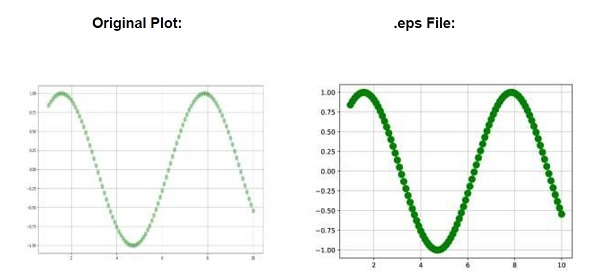# Matplotlib Plots Lose Transparency When Saving as .ps/.eps

Whenever plots are saved in .eps/.ps, then the transparency of the plots get lost.

To compare them, we can take the following Steps −

• Create x_data and y_data using numpy.

• Plot x_data and y_data (Step 1), using the plot() method, with less aplha value, to make it more transparent.

• Use the grid() method to prove the transparency of the line.

• Save the created plot in .eps format.

• To display the figure, use the show() method.

## Example

import numpy as np
from matplotlib import pyplot as plt
plt.rcParams["figure.figsize"] = [7.50, 3.50]
plt.rcParams["figure.autolayout"] = True
x_data = np.linspace(1, 10, 100)
y_data = np.sin(x_data)
plt.plot(x_data, y_data, c='green', marker='o', alpha=.35, ms=10, lw=1)
plt.grid()
plt.savefig("lost_transparency_img.eps")
plt.show()

## Output

The PostScript backend does not support transparency; partially transparent artists will be rendered opaque.